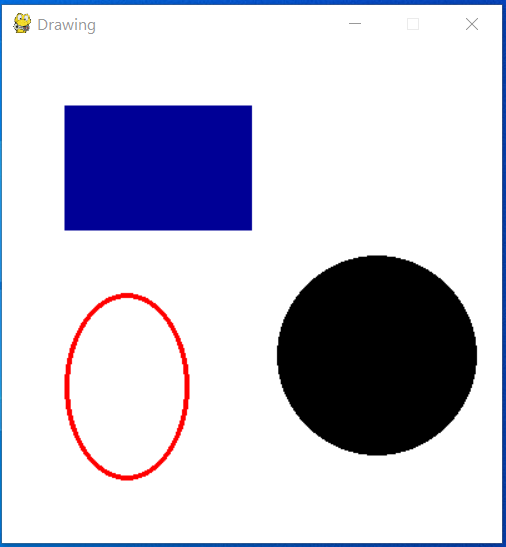# Python - Drawing different shapes on PyGame window

Pygame is a multimedia library for Python for making games and multimedia applications. In this article we will see how to use the pygame module to draws many different shapes on the screen taking into consideration, its height, width and position in the pygame window.

In the below program we initialize the pygame moduel and then deifne the colour and dimension for the image. Next we add the different shapes as per the syntax and carefully mention the arguments to the darw functions so that the images do not overlap with each other. The screen.blit function paints the screen while the while loop keeps listening the end of the game is clicked.

## Example

import pygame
pygame.init()
# define the RGB value
white = (255, 255, 255)
green = (0, 255, 0)
blue = (0, 0, 150)
black = (0, 0, 0)
red = (255, 0, 0)
# assigning values to X and Y variable
X = 400
Y = 400
# create display surface
display_surface = pygame.display.set_mode((X, Y))
# set the pygame window name
pygame.display.set_caption('Drawing')
# fill the surface object
display_surface.fill(white)
# draw a circle using draw.circle()
pygame.draw.circle(display_surface,
black, (300, 250), 80, 0)
# draw a ellipse using draw.ellipse()
pygame.draw.ellipse(display_surface, red,
(50, 200, 100, 150), 4)
# draw a rectangle using draw.rect()
pygame.draw.rect(display_surface, blue,
(50, 50, 150, 100))
# infinite loop
while True:
# iterate over the list of Event
for event in pygame.event.get():
# if event object type is QUIT
if event.type == pygame.QUIT:
# deactivates the pygame library
pygame.quit()
# quit the program.
quit()
pygame.display.update()

## Output

Running the above code gives us the following result −